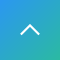1. HOME
2. Research
3. Communication Science Tanaka-Ishii Laboratory

# Communication Science Tanaka-Ishii Laboratory

Mathematical/Computational Exploration of Social Complex Systems

We explore the universal properties underlying large scale social systems through mathematical models derived by computing with big data obtained from large-scale resources. Using these models, we explore new ways of engineering to aid human social activities.

#### Analysis of large-scale social systems by applying complex systems theory

Common scaling properties are known to hold across various largescale social systems. Using real, large-scale data, we study the nature of these properties from the viewpoints such as complexity, degree of fluctuation, and self-similarity, and construct a mathematical model that explains them.

#### Deep/Machine learning methods for complex systems

We discuss the potential and limitations of deep learning and other machine learning techniques with respect to the nature of complex systems, and we study directions for improvement. Moreover, we explore unsupervised and semi-supervised methods for state-of-theart learning techniques.

#### Mathematical informatics across language, financial markets, and communication

We explore common universal properties underlying language, finance, and communication, through computing with various kinds of large-scale data, and we apply our understanding of those properties to engineering across domains. For example, we study financial market analysis by using blogs and other information sources, and we simulate information spread on a large-scale communication network.Box plots of the Taylor exponents for different kinds of dataTaylor's Law results for a time series sample produced by a Markov model (left) and a state-of the art neural model (middle), and a human text (right)

#### Member

•• Specialized field：Computational/mathematical linguistics, Deep learning and machine learning, Mathematical modeling of time series and networks, Complex systems theory for discrete systems
＜As of May 2020＞

Tags